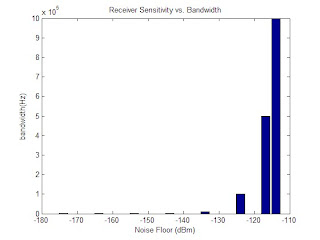## Friday, April 16, 2010

### Determining receiver sensitivity using kTB, NF, and S/N requirementReceiver sensitivity is the minimum input signal required to produce a required signal to noise ratio. In other words, it answers the common question in virtually any communication system "Is my signal good enough?"

Many system specifics are based on receiver sensitivity. For example, in a cellular system it can help you determine "good" coverage from a cell tower antenna. In digital TV receiver sensitivity can be used to determine if you need an antenna on your house to have a 'good' signal.

In test and measurement, receiver sensitivity is used to determine what is required to make a 'good' (accurate & repeatable) measurement. If you know the receiver sensitivity for a spectrum analyzer for example, you can make judgments about the quality of measurements and how to best setup the measurement.

Calculating rx_sens (receiver sensitivity) is pretty straight forward.

Step 1: Calculate kTB (noise floor) for the bandwidth of interest
where:
k = Boltzmann constant (1.38*10E-23)
T = temperature in Kelvin
B = bandwidth of interest in Hertz

Step 2: Convert to dBm
30+10*log(kTB)

For example: For a bandwidth of 30KHz
example: For a signal with a bandwidth of be 30Khz
Noise Floor = kTB = 1.38E-23 * 300 * 30000 = -129dBm

Step 3: Estimate receiver senstivtiy
Rx_sens = Noise Floor + NF of the receiver + S/N required

Example: For 10dB noise figure receiver, with a 20dB S/N requirement:
RX_Sens = -129dBm + 10dB + 20dB = -99dBm

Now we know what level we need at the receiver. Stay tuned for the next post regarding link budgets. We'll see how to use this number in our link calculations to determine if the transmitted power level is 'good enough' to meet our RX_Sens requirement.

%% Receiver Sensitivity
% James Eastham
% Member, IEEE
% Revision: R1
clear all;
close all;
b = [1 10 100 1e3 10e3 100e3 500e3 1e6]; %bandwidth every decadeHz
k = 1.38E-23; %Boltzmann's constant
T = 300; %Temp
n_floor = k*T*b;
n_floor_dBm = 30 + (10*log10(n_floor));
figure('Color',[1 1 1]);
bar(n_floor_dBm,b);
ylabel('bandwidth(Hz)');
xlabel('Noise Floor (dBm)');
title('Receiver Sensitivity vs. Bandwidth');